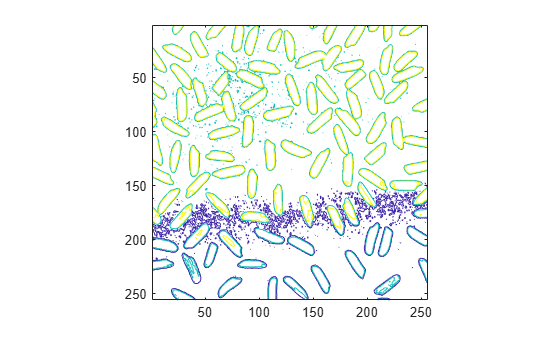imcontour

Create contour plot of image data

Description

imcontour(I) draws a contour plot of the grayscale image I, choosing the number of levels and the values of levels automatically. imcontour automatically sets up the axes so their orientation and aspect ratio match the image.

example

imcontour(I,levels) specifies the number, levels, of equally spaced contour levels in the plot.

imcontour(I,V) draws contour lines at the data values specified in vector V. The number of contour levels is equal to length(V).

imcontour(x,y,___) uses the vectors x and y to specify the image x- and y coordinates.

imcontour(___,LineSpec) draws the contours using the line type and color specified by LineSpec. Marker symbols are ignored.

[C,h] = imcontour(___) returns the contour matrix, C, and the handle, h, to the contour patches drawn onto the current axes.

Examples

collapse all

This example shows how to create a contour plot of an image.

Read grayscale image and display it. The example uses an example image of grains of rice.

imshow(I)Create a contour plot of the image using imcontour .

figure;
imcontour(I,3)Input Arguments

collapse all

Grayscale image, specified as an m-by-n matrix.

Data Types: single | double | int16 | uint8 | uint16 | logical

Number of contour levels, specified as a numeric scalar.

Value of contour levels, specified as a numeric vector with length greater than or equal to two. Use V = [v v] to compute a single contour at level v.

Image x values, specified as one of the following:

• 2-element numeric vector of the form [xmin xmax] — Image extent in the x direction.

• n-element numeric vector — x-coordinate of each column.

Image y values, specified as one of the following:

• 2-element numeric vector of the form [ymin ymax] — Image extent in the y direction.

• m-element numeric vector — y-coordinate of each row.

Line style and color, specified as a character vector or string containing a line style specifier, a color specifier, or both.

Example: '--r' specifies red dashed lines

These two tables list the line style and color options.

Line StyleDescriptionResulting Line
'-'Solid line'--'Dashed line':'Dotted line'-.'Dash-dotted lineColor SpecifierDescription

y

yellow

m

magenta

c

cyan

r

red

g

green

b

blue

w

white

k

black

Output Arguments

collapse all

Contour matrix, returned as a matrix with two rows. The matrix is defined according to the ContourMatrix property of the Contour object, h.

Contour patches, returned as a handle to a Contour object.